### Home > MC1 > Chapter 7 > Lesson 7.3.2 > Problem7-116

7-116.

Copy and complete each of the Diamond Problems below. The pattern used in the Diamond Problems is shown at right.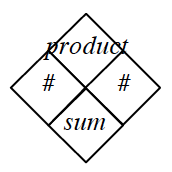1.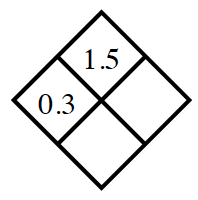Refer to problem 6-121 for help on this problem.

1.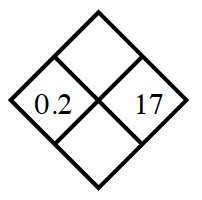Add $x$ and $y$ to fill the sum box. Multiply them to fill the product box.

1.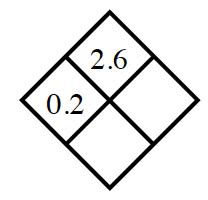What times $0.2$ is equal to $2.6$?

$y = 13$. Can you find the sum of $0.2$ and $13$?

1.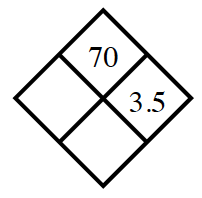This problem is very similar to part (c).